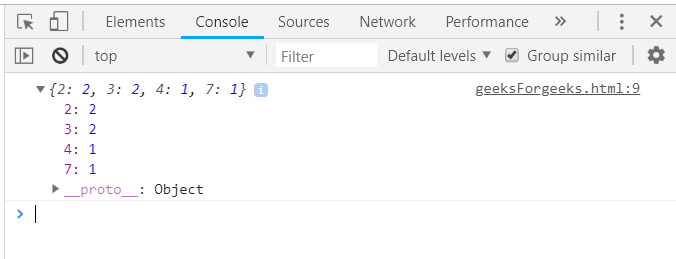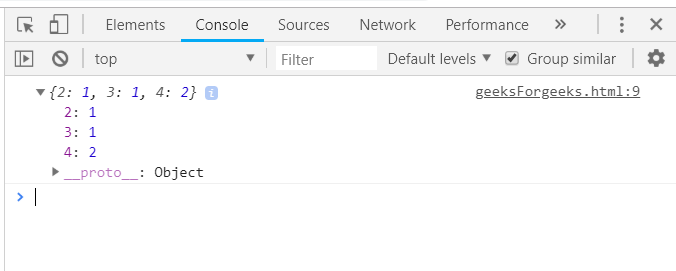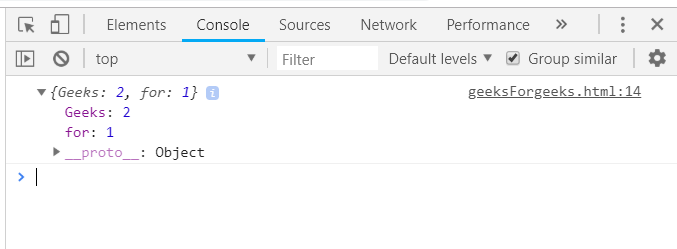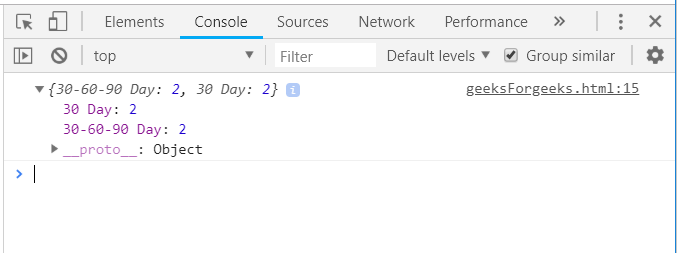Related Articles
Underscore.js | countBy() with Examples
• Last Updated : 14 Feb, 2019

The Underscore.js is a JavaScript library that provides a lot of useful functions that helps in the programming in a big way like the map, filter, invoke etc even without using any built-in objects.
The _.countBy() function is used to sort a list into groups and returns a count for the number of objects in each group. It works by matching the value of each element to the other. If they matches then the count of one collection increases by 1 otherwise the count of another collections/groups which has that value increases by 1. It can also pass a function based on who’s result will collect the elements and increase the count of each group. It can match both on the basis of number and also by string.

Syntax:

`_.countBy(list, iteratee, [context]) `

Parameters: This function accepts three parameters as mentioned above and described below:

• List: This parameter is used to hold the list of items.
• Iteratee: This parameter is used to hold the test condition.
• Context: The text content which need to display.

Return values: It returns the collections as the different arrays.

Passing Math.ceil() function to the _.countBy() function: The _.countBy() function takes the element from the list one by one and pass it to the other function mentioned here. Here the function is taking the ceil of each number and returning it’s values. So, all the value of the array are counted one by one after their ceil has been taken and then counted according to whether they are same or different.

Example:

 `<``html``> ` `    ``<``head``> ` `        ``<``script` `type``=``"text/javascript"` `src``= ` `        ``"https://cdnjs.cloudflare.com/ajax/libs/underscore.js/1.9.1/underscore.js"``> ` `        `` ` `    `` ` `    ``<``body``> ` `        ``<``script` `type``=``"text/javascript"``> ` `        ``console.log(_.countBy([2.7, 3.4, 6.6, 1.2, 2.0, 2.4], ` `                   ``function(num){ return Math.ceil(num); })); ` `        `` ` `    `` ` `                     `

Output:Using length() in the _.countBy() function: Passing the array elements to the countBy() function. Then, find out the length of each element and make collections of the lengths that are same. Finally, display the count of each collection with the respective lengths along the left.

Example:

 `<``html``> ` `    ``<``head``> ` `        ``<``script` `type``=``"text/javascript"` `src``= ` `        ``"https://cdnjs.cloudflare.com/ajax/libs/underscore.js/1.9.1/underscore.js"``> ` `        `` ` `    `` ` `    ``<``body``> ` `        ``<``script` `type``=``"text/javascript"``> ` `           ``console.log(_.countBy(['HTML', 'CSS3', 'JS', 'PHP'], 'length')); ` `        `` ` `    `` ` ` `

Output:Using one property of the array passed in the _.countBy() function: First declare the array (here array is ‘arr’). Choose one condition on which need to count like here ‘prop3’. Then the elements which have the same value in the ‘prop3’ will be grouped in 1 collection. The final result will contain the prop3 in the left side along with their count on the right. Like here in prop3, “Geeks” is coming two times, so it’s count will be 2. Console.log the final answer.

Example:

 `<``html``> ` `    ``<``head``> ` `        ``<``script` `type``=``"text/javascript"` `src``= ` `        ``"https://cdnjs.cloudflare.com/ajax/libs/underscore.js/1.9.1/underscore.js"``> ` `        `` ` `    `` ` `    ``<``body``> ` `        ``<``script` `type``=``"text/javascript"``> ` `            ``var arr =  [ ` `                ``{prop1:"10", prop2:"07", prop3: "Geeks"}, ` `                ``{prop1:"12", prop2:"86", prop3: "for"}, ` `                ``{prop1:"11", prop2:"58", prop3: "Geeks"}  ` `            ``]; ` `           ``console.log(_.countBy(arr, 'prop3')); ` `        `` ` `    `` ` ` `

Output:Passing ‘date’ as property of the array to the _.countBy() function together: First define an array with one property as ‘date’ of the format ‘dd-mm-yy’. Then pass the array and the ‘date’ property to the _.countBy() function. The elements having the same date will be grouped as one collection and then the count of each group will be displayed in the result.

Example:

 `<``html``> ` `    ``<``head``> ` `        ``<``script` `type``=``"text/javascript"` `src``= ` `        ``"https://cdnjs.cloudflare.com/ajax/libs/underscore.js/1.9.1/underscore.js"``> ` `        `` ` `    `` ` `    ``<``body``> ` `        ``<``script` `type``=``"text/javascript"``> ` `            ``var orders = [ ` `                ``{    date:"30-60-90 Day", Name:"Kim", amount:415     }, ` `                ``{   date:"30-60-90 Day", Name:"Kelly", amount:175     }, ` `                ``{   date:"30 Day", Name:"Shelly", amount:400     }, ` `                ``{   date:"30 Day", Name:"Sarvesh", amount:180     } ` `                ``]; ` `            ``console.log(_.countBy(orders, "date")); ` `        `` ` `    `` ` ` `

Output:Note: These commands will not work in Google console or in Firefox as for these additional files need to be added which they didn’t have added. So, add the given links to your HTML file and then run them.

 `<``script` `type``=``"text/javascript"` `src` `= ` `"https://cdnjs.cloudflare.com/ajax/libs/underscore.js/1.9.1/underscore-min.js"``> ` ` `My Personal Notes arrow_drop_up
Recommended Articles
Page :